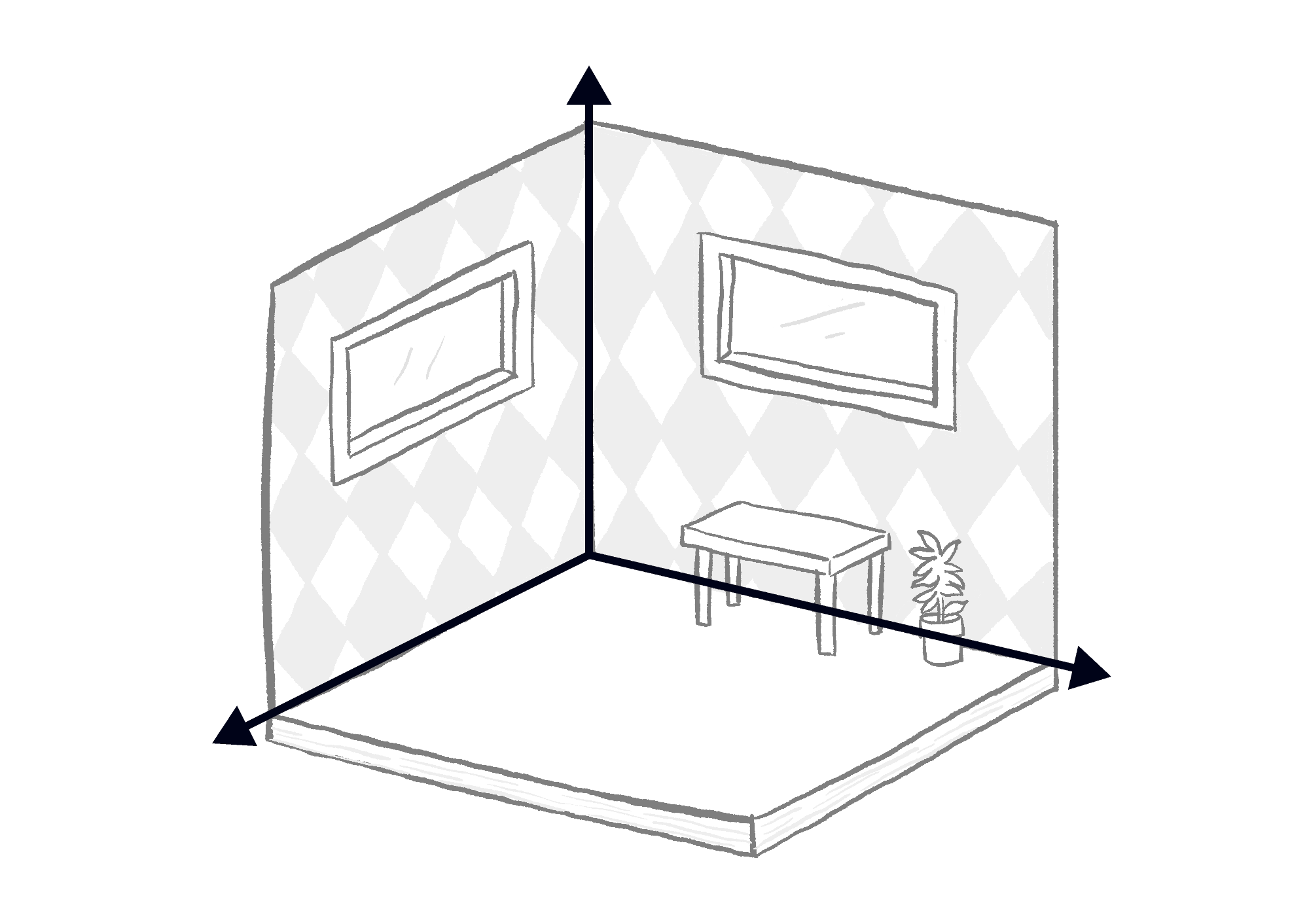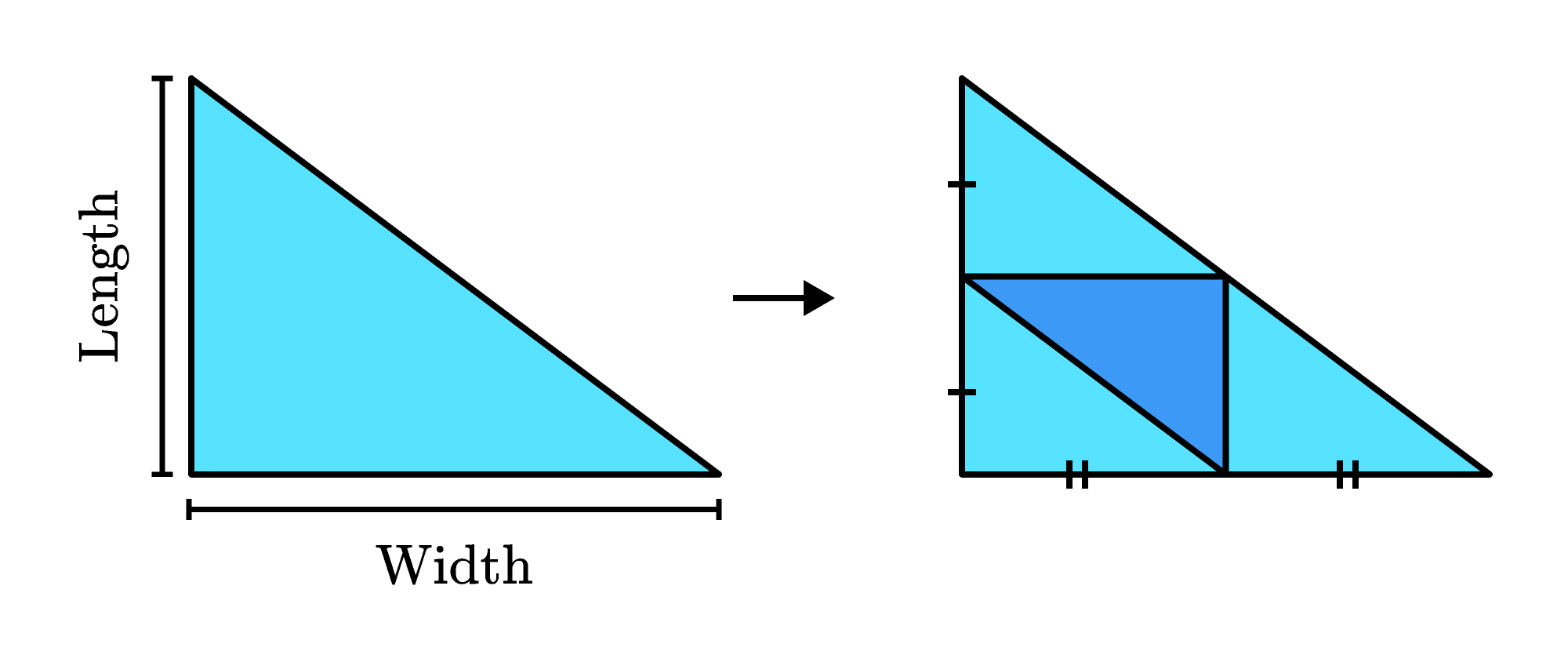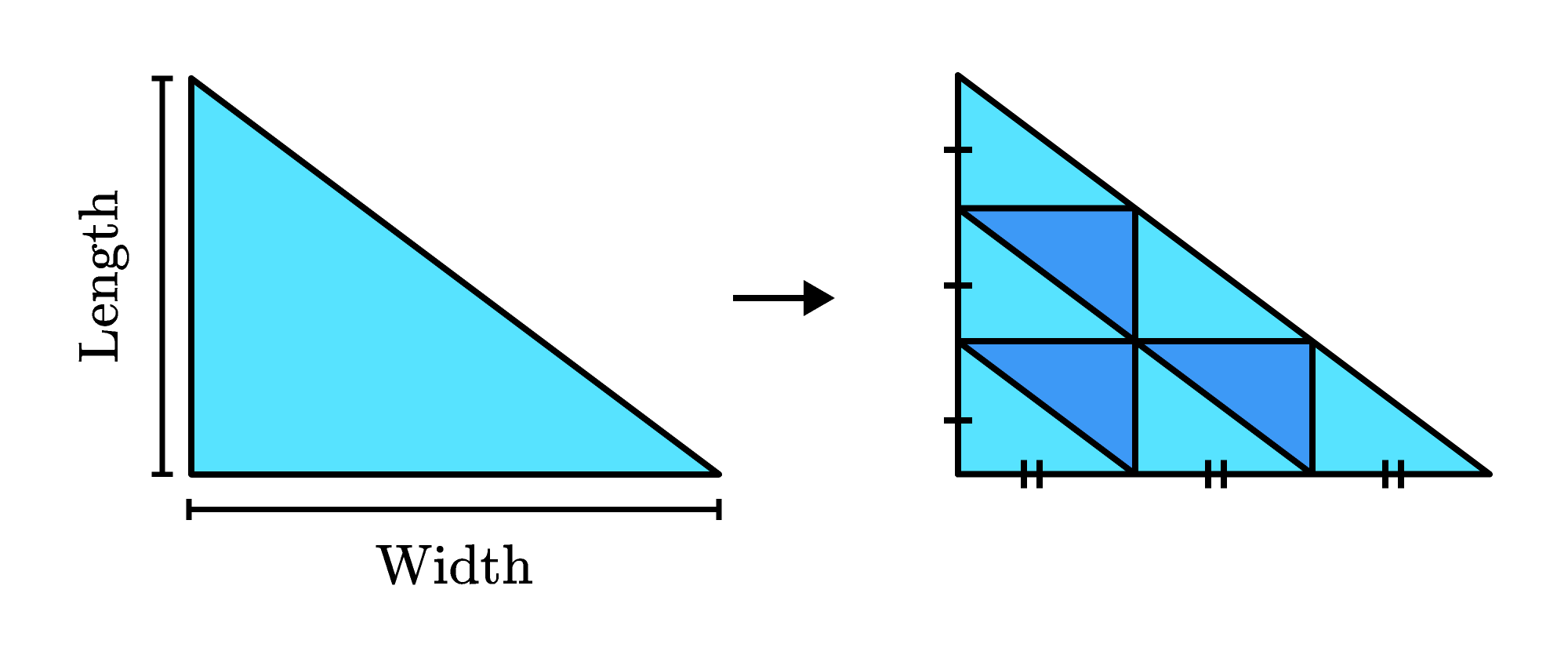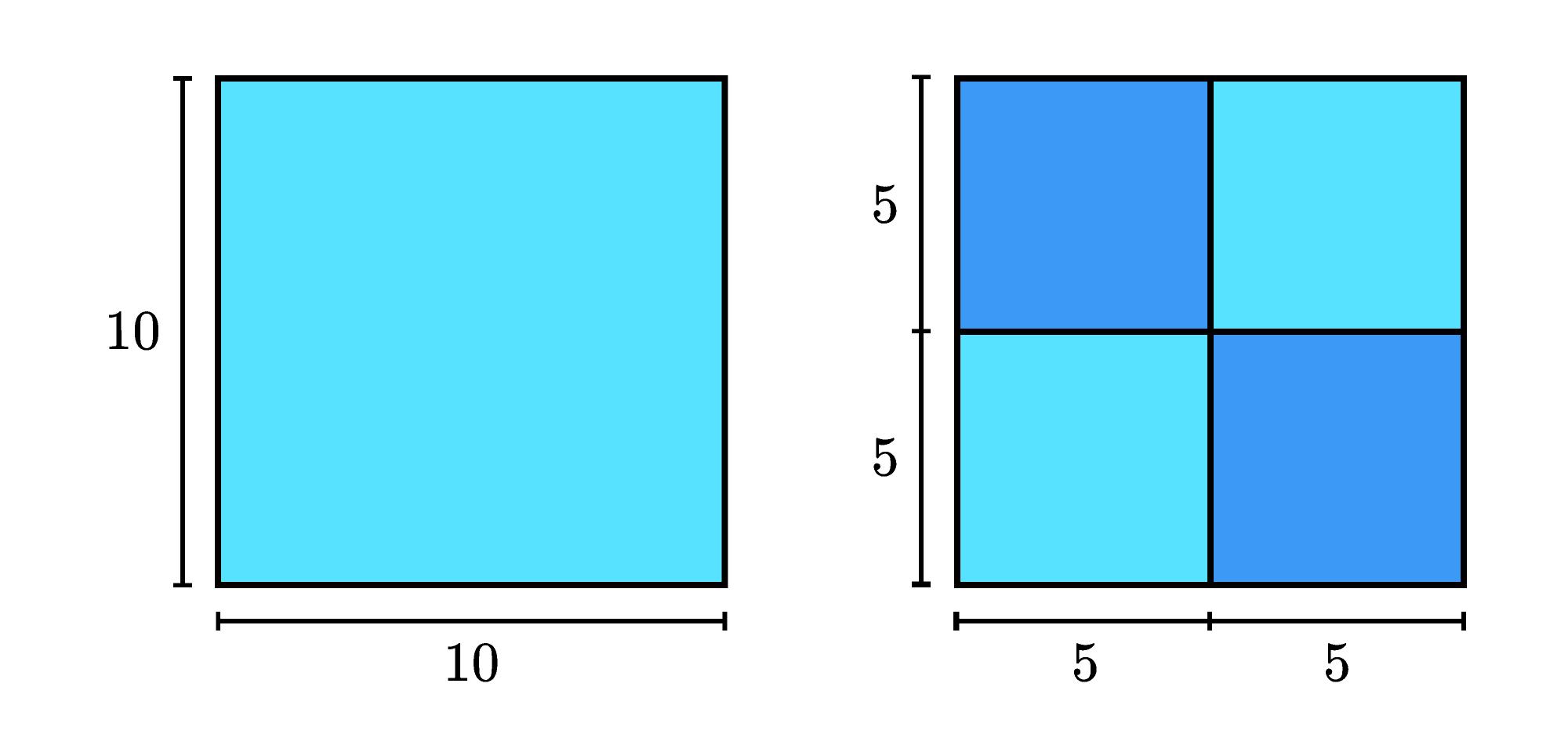## Dimensional Scaling

How many dimensions do we live in? Many would say three, some would say four — as Einstein taught us with the concept of spacetime that time is a dimension existing along with the familiar three spatial dimensions. In some theories of physics, there are thought to be $10, 11, 24,$ or $26$ dimensions! What are all these other dimensions?

But this isn’t a science problem, this is a math problem. For a look at how we define dimension geometrically, keep reading. Or jump straight to today’s challenge.

Let’s unpack the “familiar three spatial dimensions.” The dimension of an object is the number of perpendicular directions that we can use to measure distances on that object. (Confusingly, these directions are also called dimensions.)In any corner of a room, the lines where the walls meet the floor or each other show three perpendicular directions; these are the three spatial dimensions we live in. Here’s how some examples from geometry fit into those $3$ dimensions:

• A point is $0$-dimensional; it can mark a position but doesn’t take up any space.
• A line is $1$-dimensional; it only has length.
• A flat shape is $2$-dimensional; it has length and width.
• A solid figure is $3$-dimensional; it has length, width, and height.Dimension also relates to how an object can be made up of smaller copies of itself. Consider a line segment. It’s a portion of a line, so it’s $1$-dimensional.

If we scale its length down by a factor of $2,$ we can put $2$ copies of the line segment together to recreate the original line segment:If we scale its length down by a factor of $3,$ we can put $3$ copies of the line segment together to recreate the original line segment:Now, instead, let's look at a right triangle. It’s a flat shape, so it's $2$-dimensional.

If we scale both its length and width down by a factor of $2,$ we can put $4$ copies of the triangle together to recreate the original triangle:If we scale both its length and width down by a factor of $3,$ we can put $9$ copies of the triangle together to recreate the original triangle:What is the relationship between dimension, scale, and the number of copies here? It’s your job to find it in today’s challenge.

# Today's Challenge

When we have a $D$-dimensional object and scale it down by a factor of $S$ in every direction, we can put $C$ copies together to recreate the original object.For example, if the object is a square, it has length and width, so its dimension is $D=2.$ Suppose we scale it down by a factor of $S=2.$ Then we can put $C=4$ copies of the scaled-down square together to recreate the original.

Which of the following equations relates $D, S,$ and $C?$

Hint: Also try considering a different scale-down factor and/or a $3$-dimensional object.